Скачать презентацию I M P A C T in Mathematics

4e5e36d4707b61f2fcc287b9c878f75b.ppt

• Количество слайдов: 41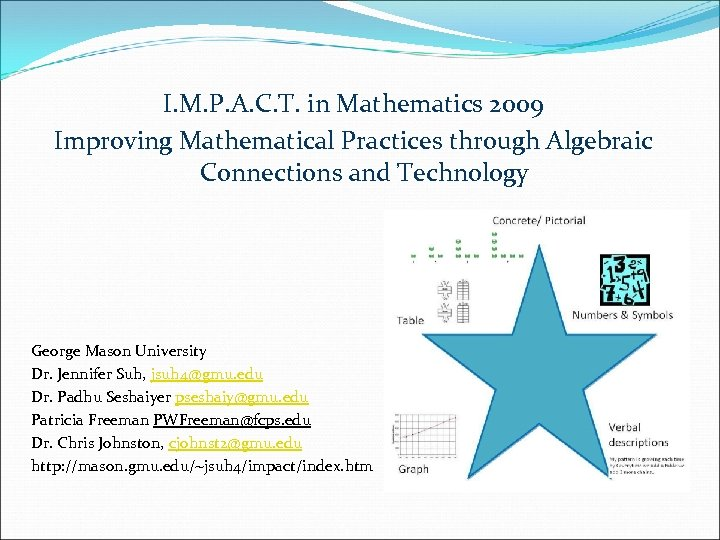I. M. P. A. C. T. in Mathematics 2009 Improving Mathematical Practices through Algebraic Connections and Technology George Mason University Dr. Jennifer Suh, jsuh [email protected] edu Dr. Padhu Seshaiyer [email protected] edu Patricia Freeman [email protected] edu Dr. Chris Johnston, cjohnst [email protected] edu http: //mason. gmu. edu/~jsuh 4/impact/index. htm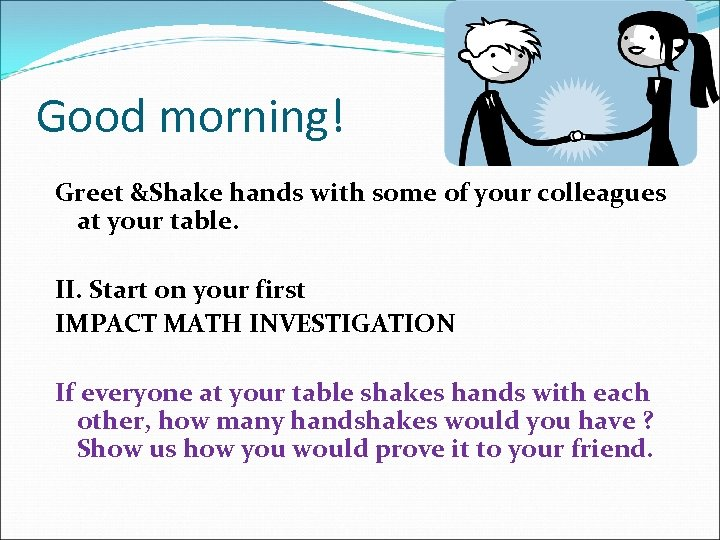Good morning! Greet &Shake hands with some of your colleagues at your table. II. Start on your first IMPACT MATH INVESTIGATION If everyone at your table shakes hands with each other, how many handshakes would you have ? Show us how you would prove it to your friend.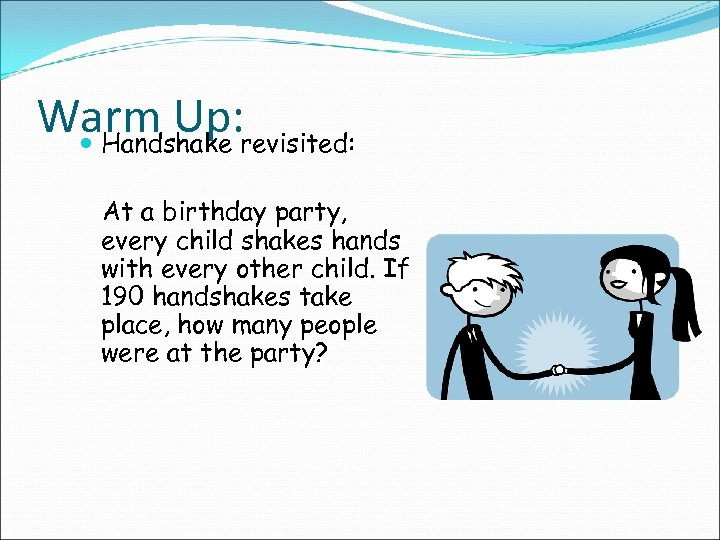Warm Up: revisited: Handshake At a birthday party, every child shakes hands with every other child. If 190 handshakes take place, how many people were at the party?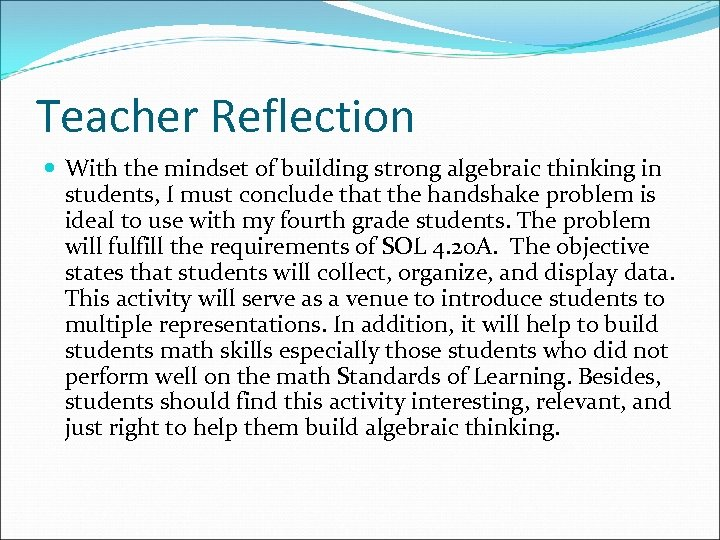Teacher Reflection With the mindset of building strong algebraic thinking in students, I must conclude that the handshake problem is ideal to use with my fourth grade students. The problem will fulfill the requirements of SOL 4. 20 A. The objective states that students will collect, organize, and display data. This activity will serve as a venue to introduce students to multiple representations. In addition, it will help to build students math skills especially those students who did not perform well on the math Standards of Learning. Besides, students should find this activity interesting, relevant, and just right to help them build algebraic thinking.Teacher Reflection After organizing the data in a table it was possible to find the rule. The rule written in the form of a formula is used to find a given number to determine the handshakes. The rule consists of Symbolic representation n(n-1)/2=h where n is the number of people shaking hands and h the total number of handshakes. The 1 is subtracted from the number since there will be no handshake by one person. When the class consists of 20 students the rule makes it easier to calculate the number of handshakes rather than drawing pictures or filling in the numbers in a chart. Over all, the formula shortens the continuous computation of numbers. For example, n(n-1)/2=h 20(20 -1) =h 20 (19) =h 380/2 =h When there are 20 students there are 190 handshakes.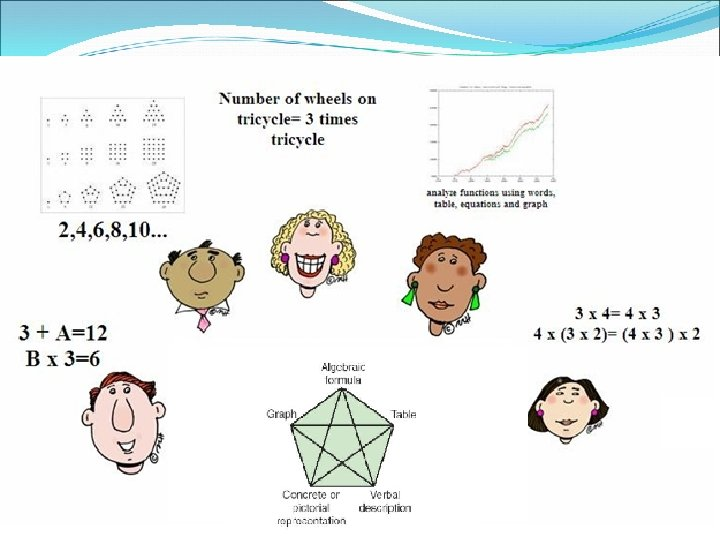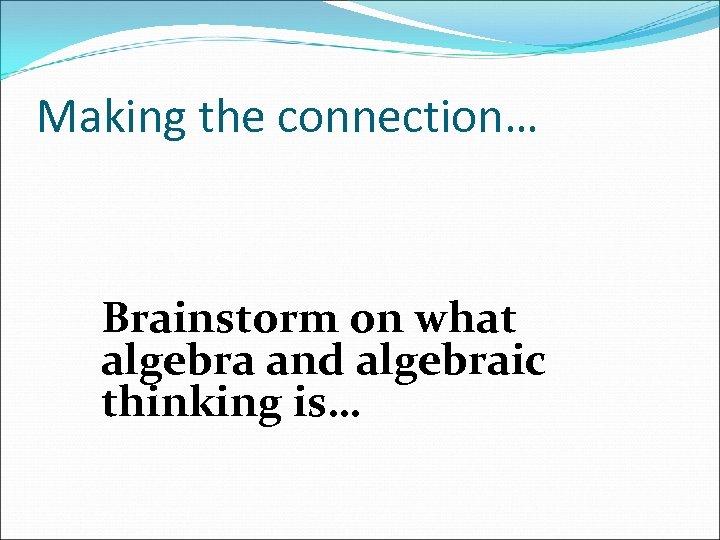Making the connection… Brainstorm on what algebra and algebraic thinking is…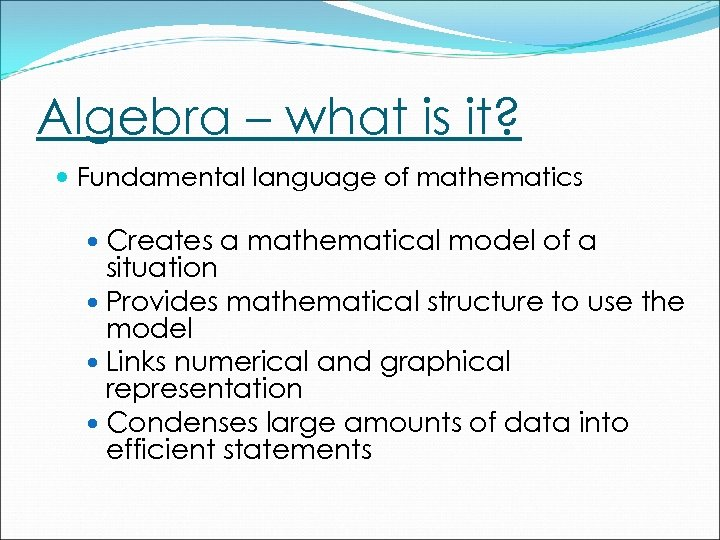Algebra – what is it? Fundamental language of mathematics Creates a mathematical model of a situation Provides mathematical structure to use the model Links numerical and graphical representation Condenses large amounts of data into efficient statements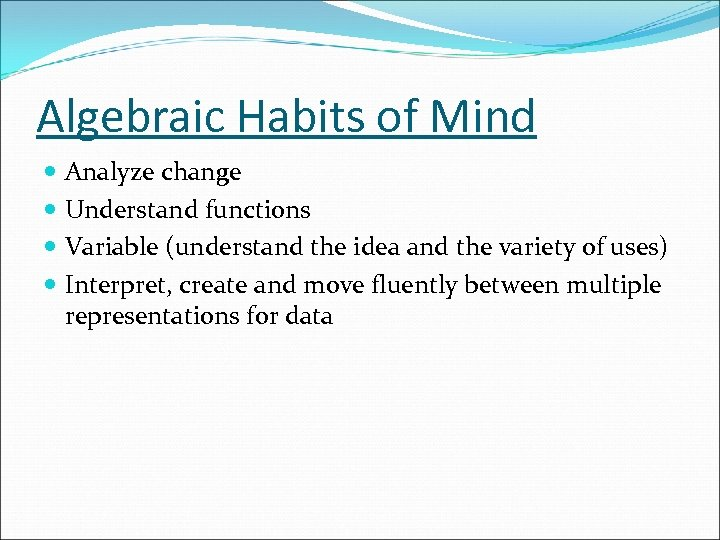Algebraic Habits of Mind Analyze change Understand functions Variable (understand the idea and the variety of uses) Interpret, create and move fluently between multiple representations for data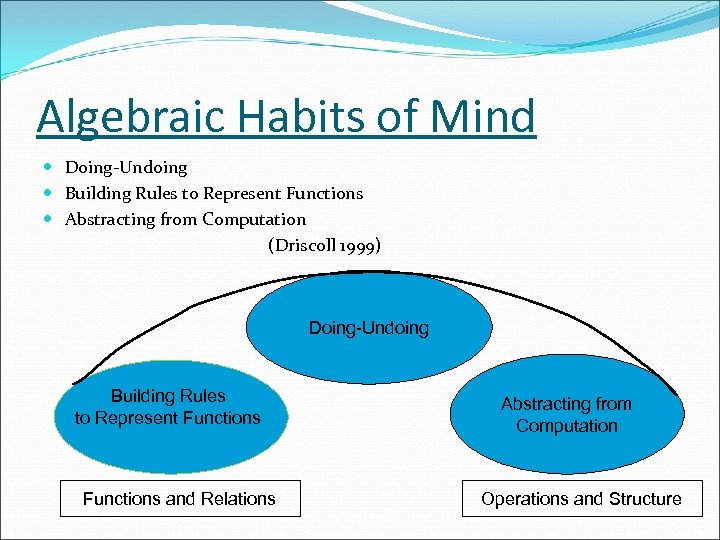Algebraic Habits of Mind Doing-Undoing Building Rules to Represent Functions Abstracting from Computation (Driscoll 1999) Doing-Undoing Building Rules to Represent Functions and Relations Abstracting from Computation Operations and Structure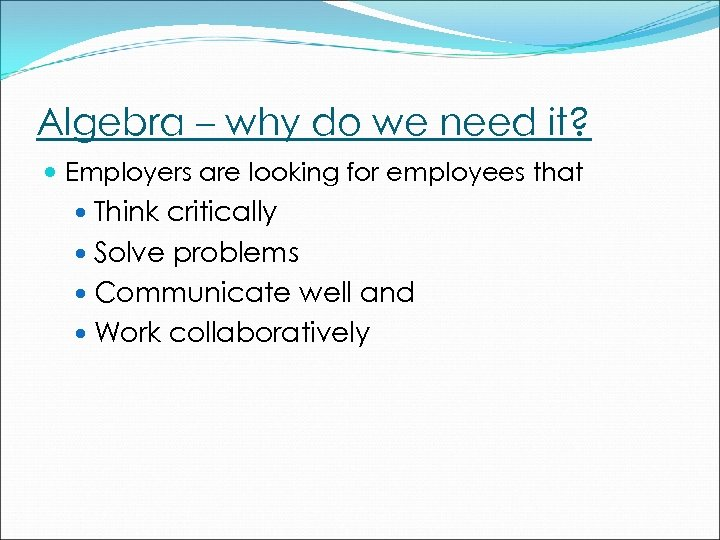Algebra – why do we need it? Employers are looking for employees that Think critically Solve problems Communicate well and Work collaboratively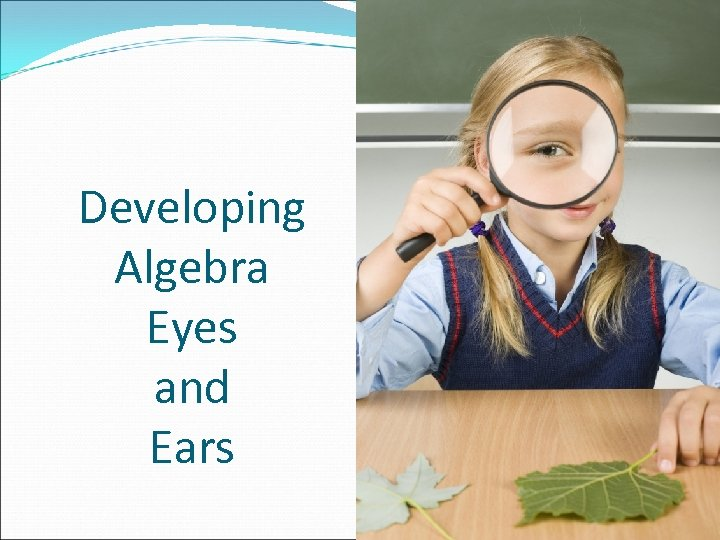Developing Algebra Eyes and Ears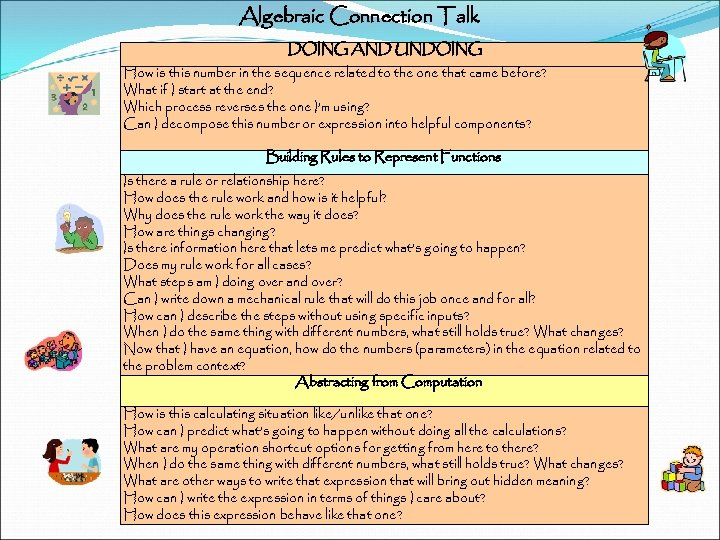Algebraic Connection Talk DOING AND UNDOING How is this number in the sequence related to the one that came before? What if I start at the end? Which process reverses the one I’m using? Can I decompose this number or expression into helpful components? Building Rules to Represent Functions Is there a rule or relationship here? How does the rule work and how is it helpful? Why does the rule work the way it does? How are things changing? Is there information here that lets me predict what’s going to happen? Does my rule work for all cases? What steps am I doing over and over? Can I write down a mechanical rule that will do this job once and for all? How can I describe the steps without using specific inputs? When I do the same thing with different numbers, what still holds true? What changes? Now that I have an equation, how do the numbers (parameters) in the equation related to the problem context? Abstracting from Computation How is this calculating situation like/unlike that one? How can I predict what’s going to happen without doing all the calculations? What are my operation shortcut options for getting from here to there? When I do the same thing with different numbers, what still holds true? What changes? What are other ways to write that expression that will bring out hidden meaning? How can I write the expression in terms of things I care about? How does this expression behave like that one?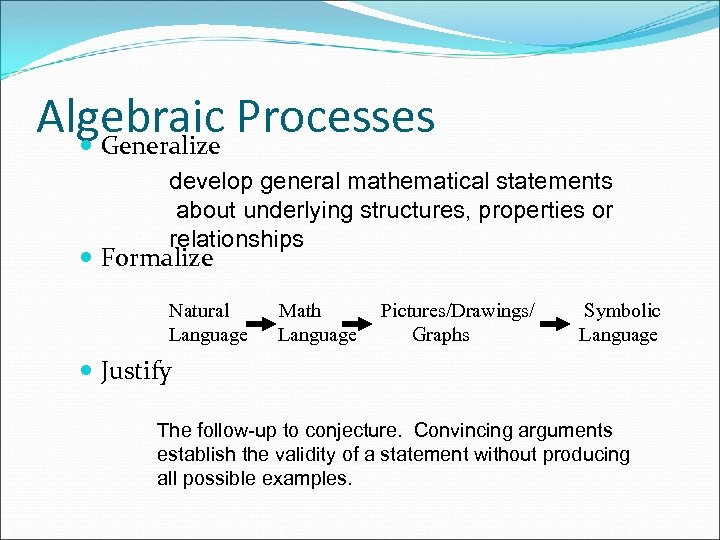Algebraic Processes Generalize develop general mathematical statements about underlying structures, properties or relationships Formalize Natural Language Math Language Pictures/Drawings/ Graphs Symbolic Language Justify The follow-up to conjecture. Convincing arguments establish the validity of a statement without producing all possible examples.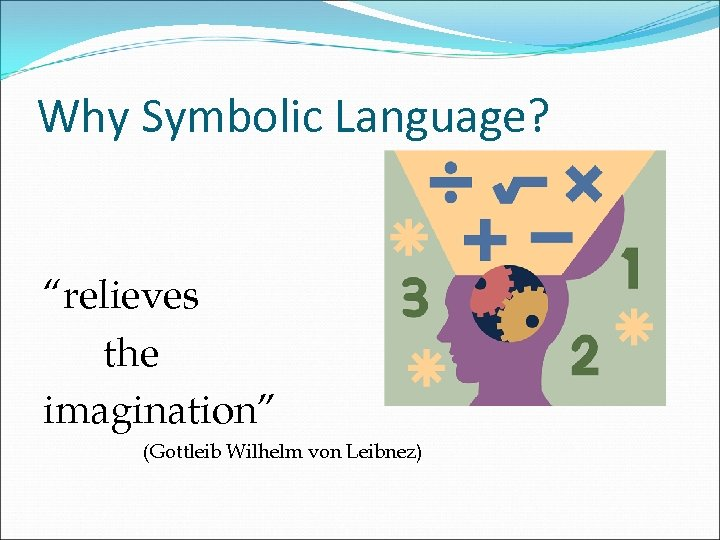Why Symbolic Language? “relieves the imagination” (Gottleib Wilhelm von Leibnez)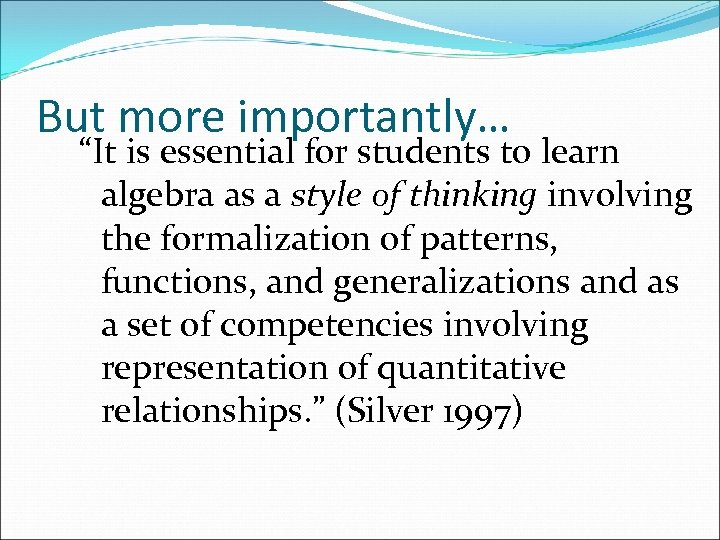But more importantly… “It is essential for students to learn algebra as a style of thinking involving the formalization of patterns, functions, and generalizations and as a set of competencies involving representation of quantitative relationships. ” (Silver 1997)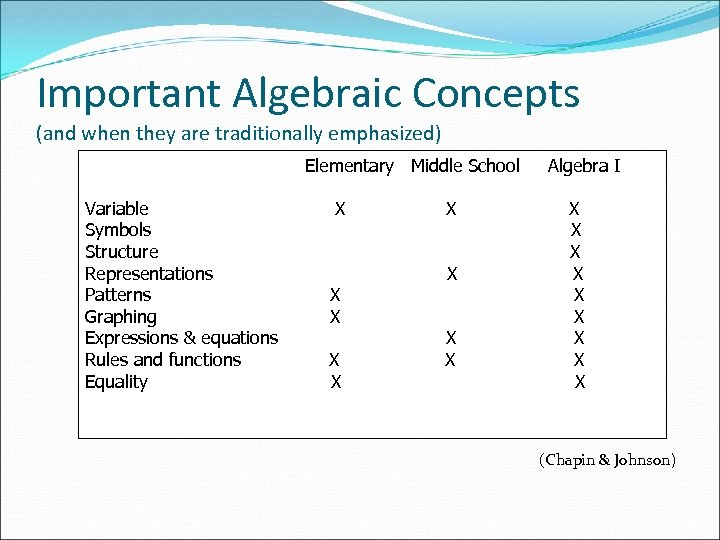Important Algebraic Concepts (and when they are traditionally emphasized) Elementary Middle School Variable Symbols Structure Representations Patterns Graphing Expressions & equations Rules and functions Equality X X X X X Algebra I X X X X X (Chapin & Johnson)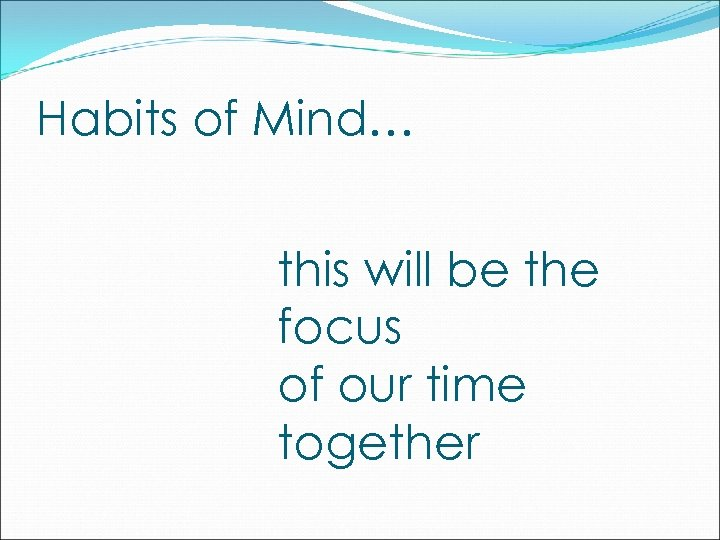Habits of Mind… this will be the focus of our time together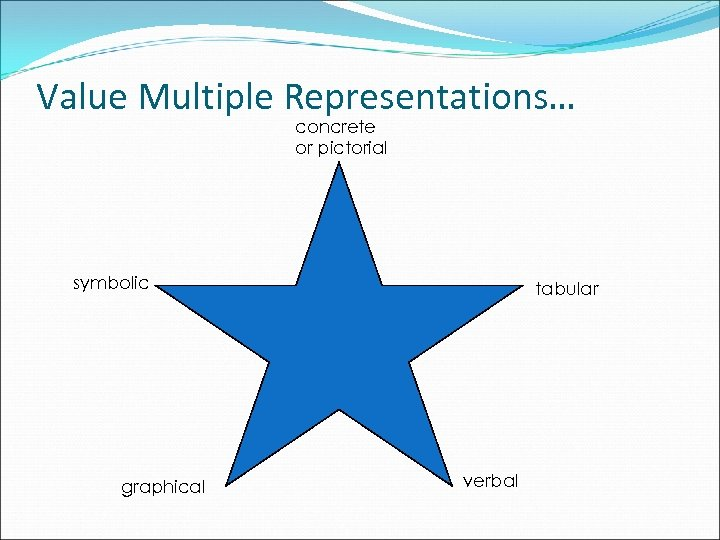Value Multiple Representations… concrete or pictorial symbolic graphical tabular verbal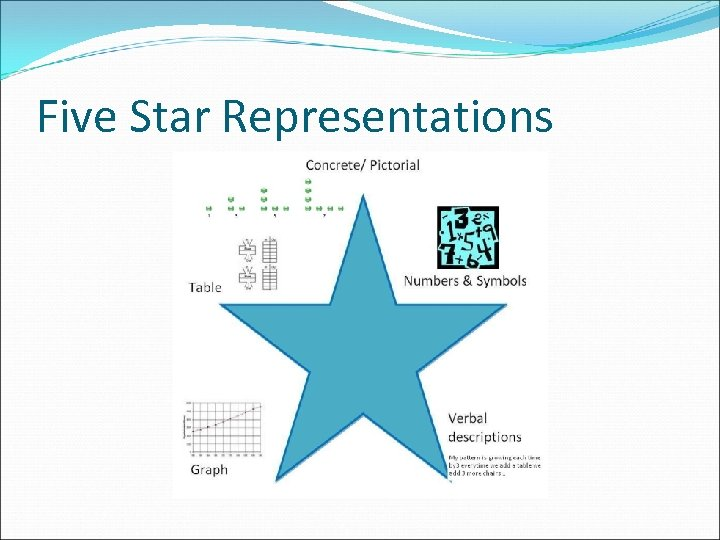Five Star Representations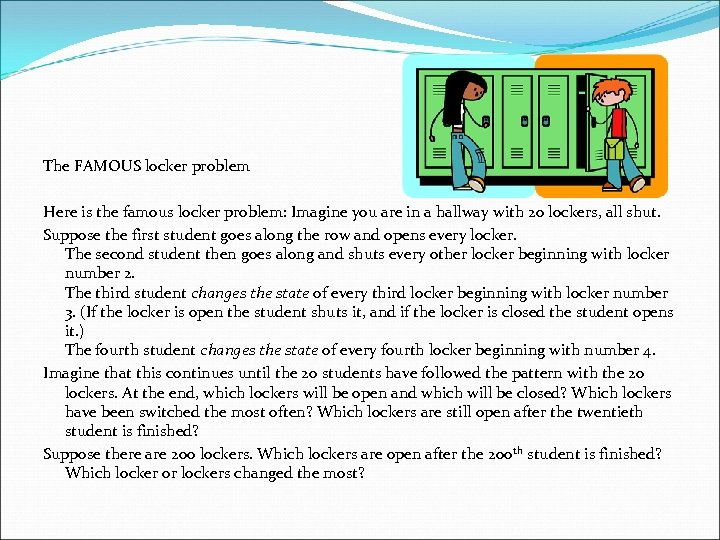The FAMOUS locker problem Here is the famous locker problem: Imagine you are in a hallway with 20 lockers, all shut. Suppose the first student goes along the row and opens every locker. The second student then goes along and shuts every other locker beginning with locker number 2. The third student changes the state of every third locker beginning with locker number 3. (If the locker is open the student shuts it, and if the locker is closed the student opens it. ) The fourth student changes the state of every fourth locker beginning with number 4. Imagine that this continues until the 20 students have followed the pattern with the 20 lockers. At the end, which lockers will be open and which will be closed? Which lockers have been switched the most often? Which lockers are still open after the twentieth student is finished? Suppose there are 200 lockers. Which lockers are open after the 200 th student is finished? Which locker or lockers changed the most?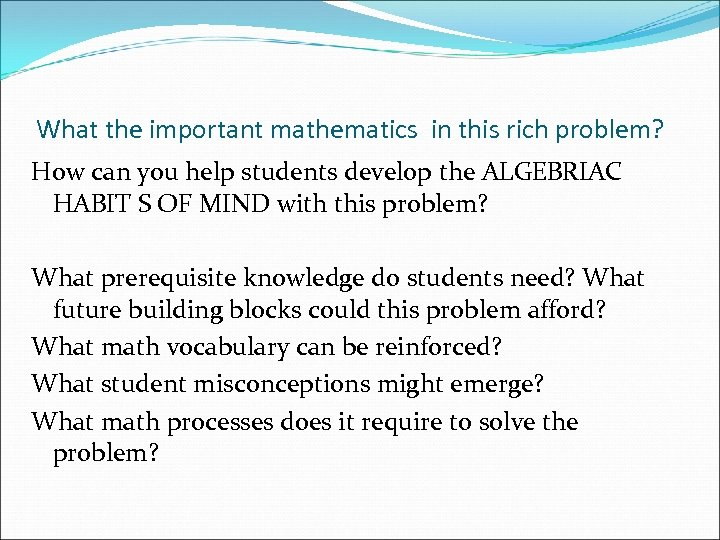What the important mathematics in this rich problem? How can you help students develop the ALGEBRIAC HABIT S OF MIND with this problem? What prerequisite knowledge do students need? What future building blocks could this problem afford? What math vocabulary can be reinforced? What student misconceptions might emerge? What math processes does it require to solve the problem?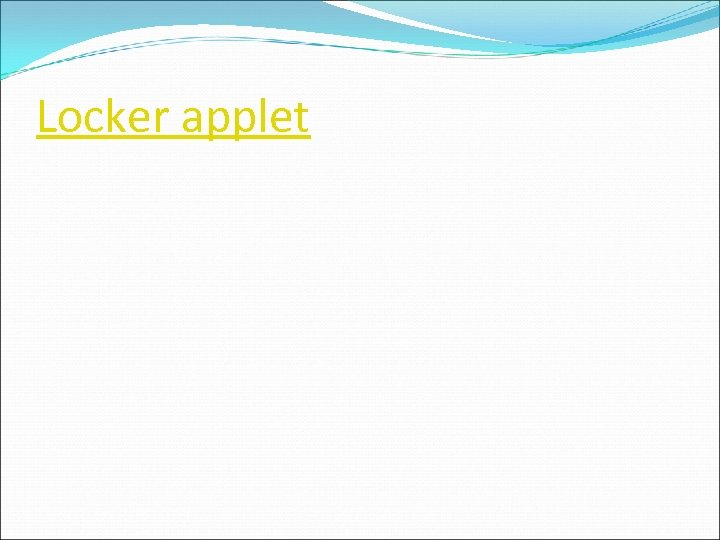Locker applet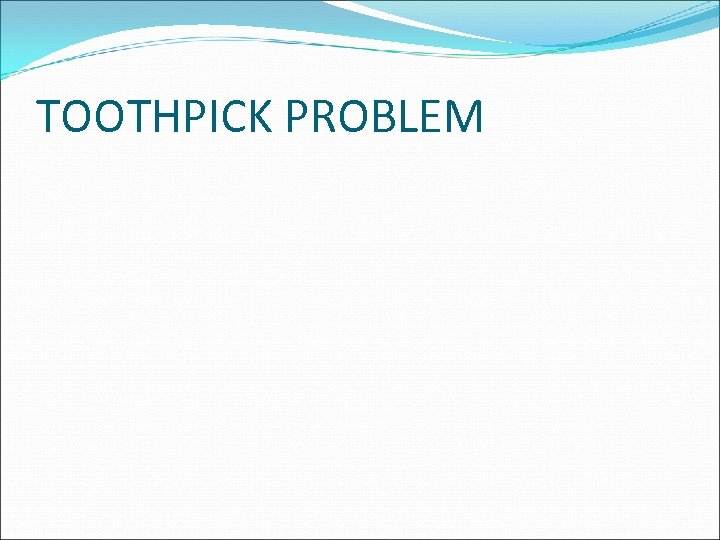TOOTHPICK PROBLEM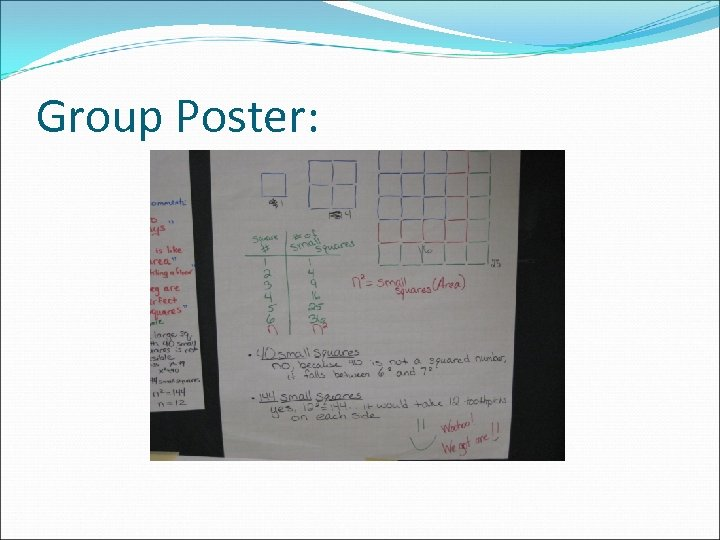Group Poster: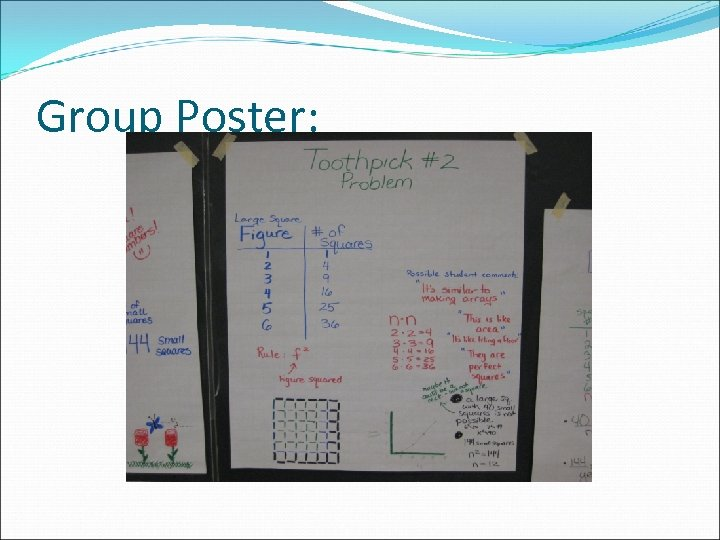Group Poster: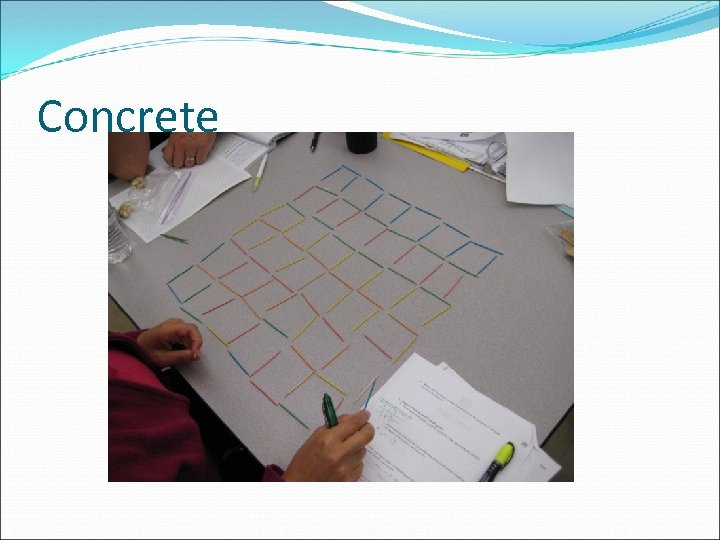Concrete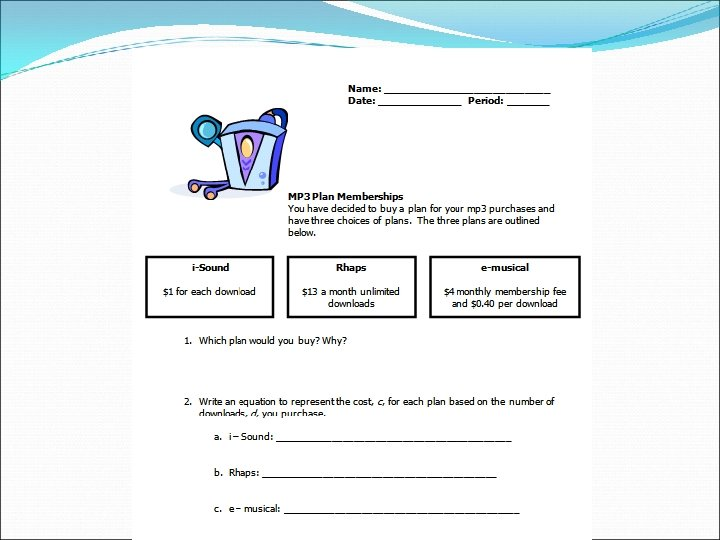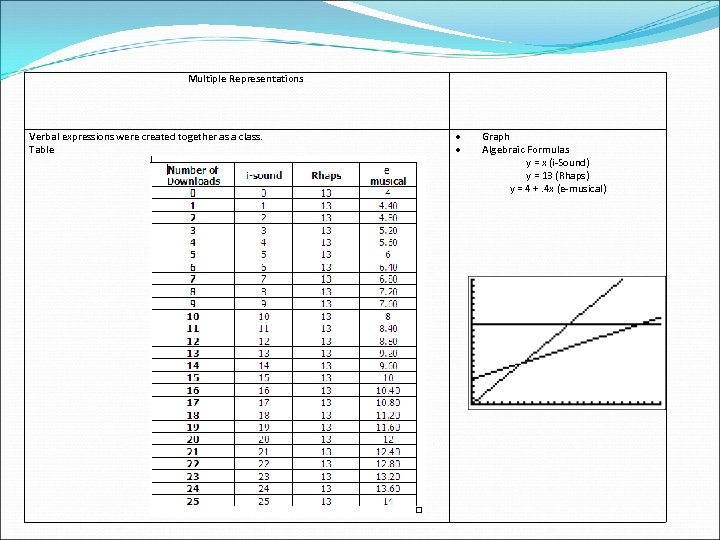Multiple Representations Verbal expressions were created together as a class. Table Graph Algebraic Formulas y = x (i-Sound) y = 13 (Rhaps) y = 4 +. 4 x (e-musical)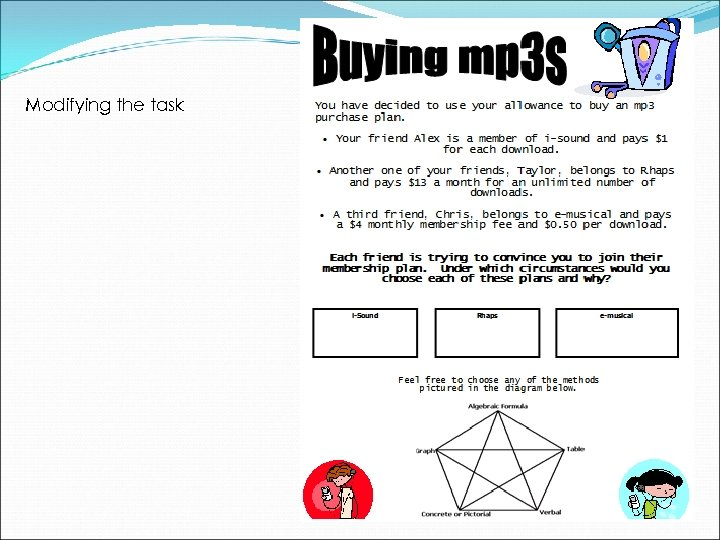Modifying the task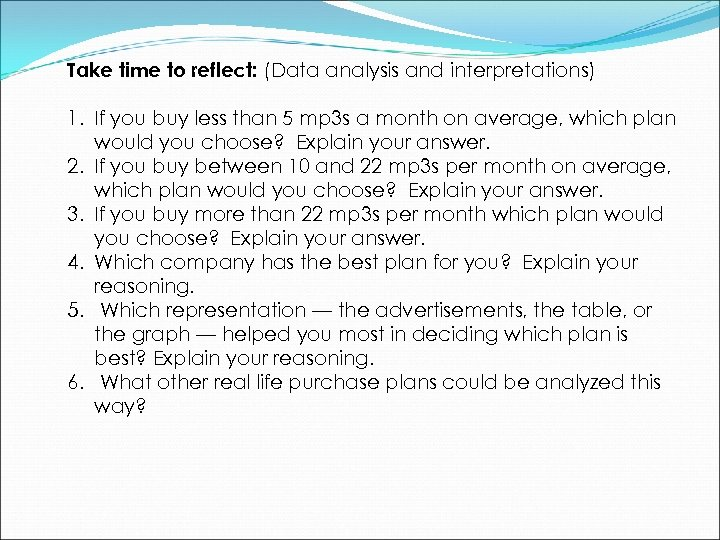Take time to reflect: (Data analysis and interpretations) 1. If you buy less than 5 mp 3 s a month on average, which plan would you choose? Explain your answer. 2. If you buy between 10 and 22 mp 3 s per month on average, which plan would you choose? Explain your answer. 3. If you buy more than 22 mp 3 s per month which plan would you choose? Explain your answer. 4. Which company has the best plan for you? Explain your reasoning. 5. Which representation — the advertisements, the table, or the graph — helped you most in deciding which plan is best? Explain your reasoning. 6. What other real life purchase plans could be analyzed this way?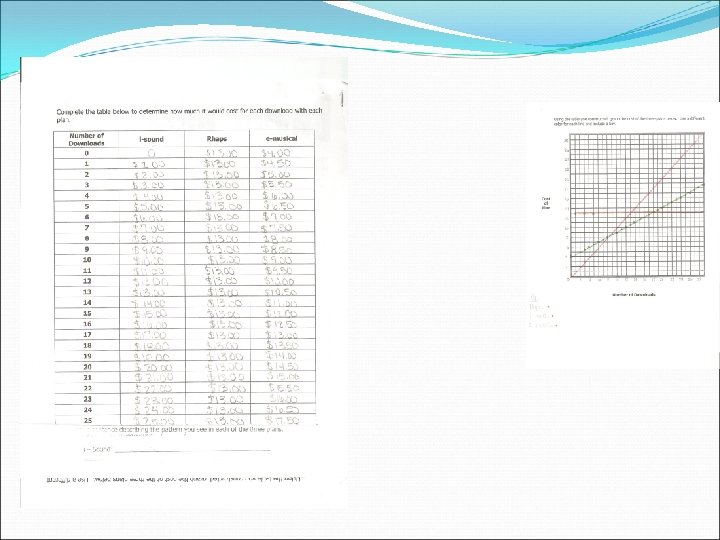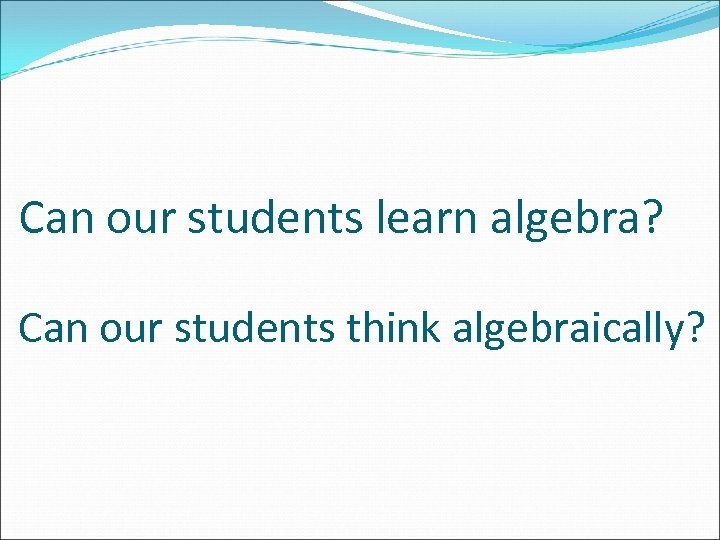Can our students learn algebra? Can our students think algebraically?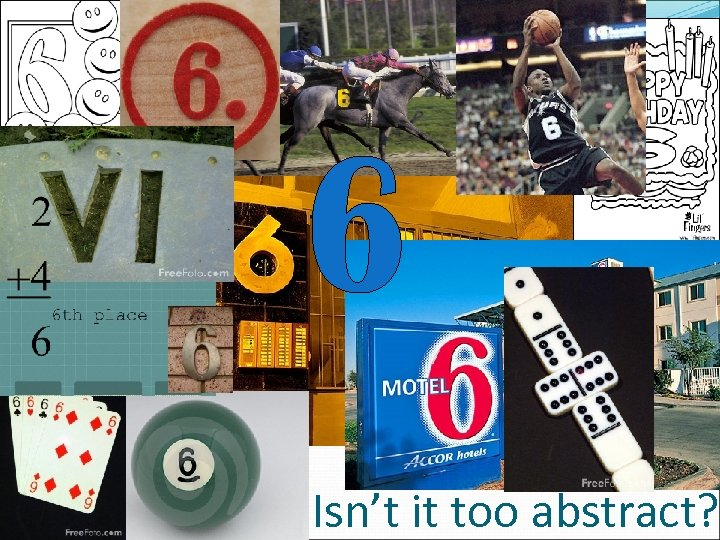Isn’t it too abstract?Isn’t it too abstract?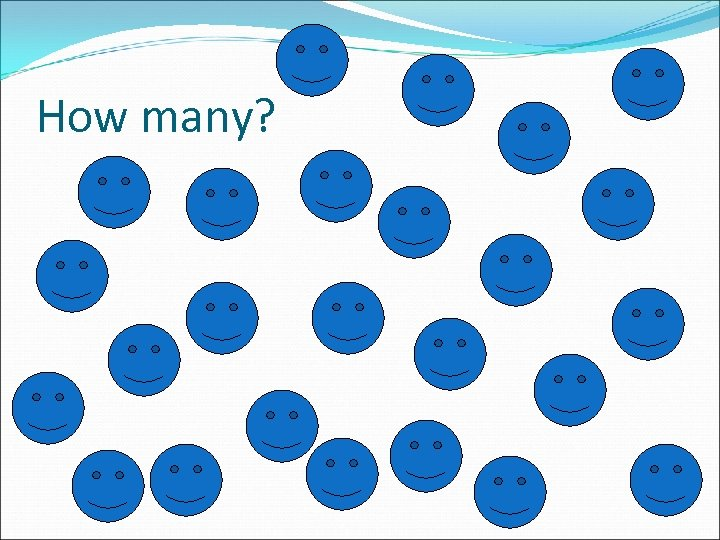How many?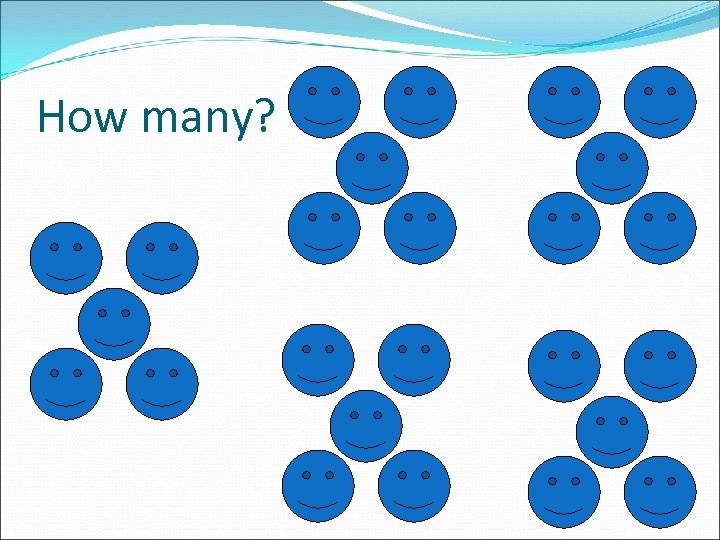How many?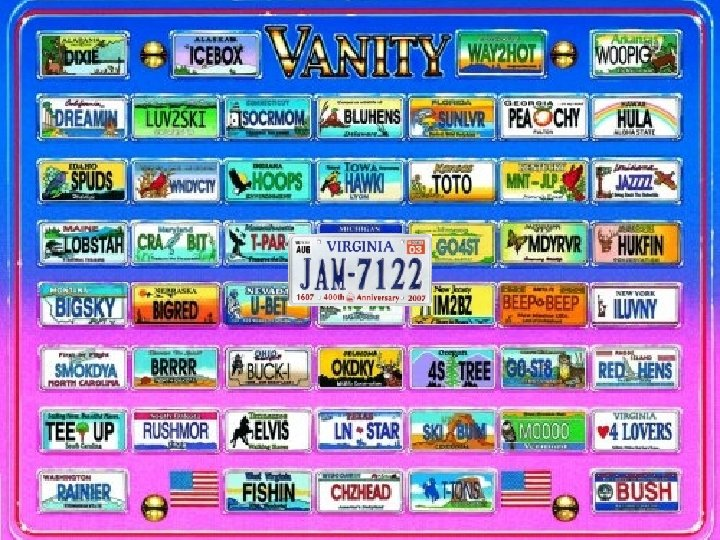We are naturally drawn to patterns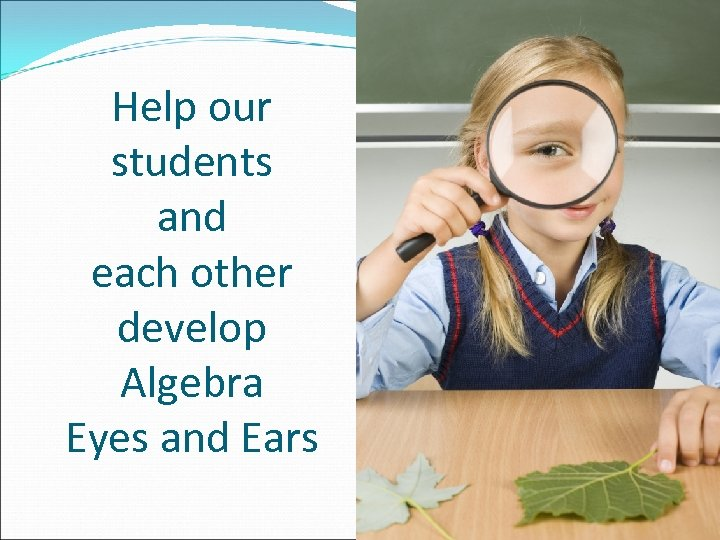Help our students and each other develop Algebra Eyes and Ears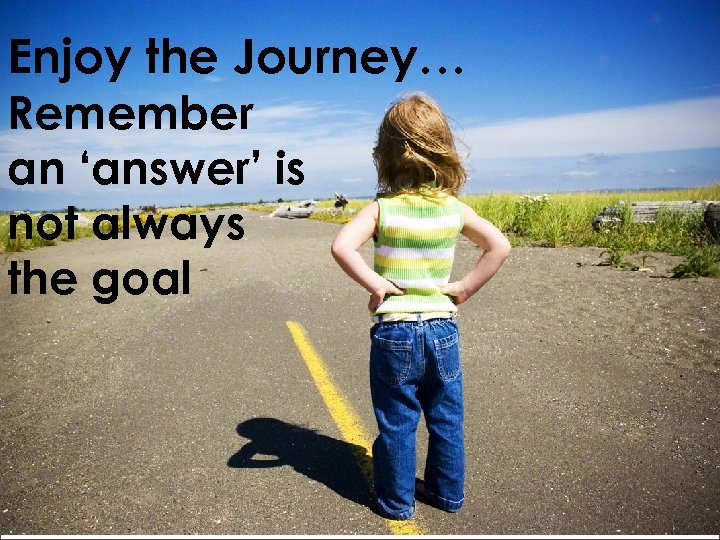Enjoy the Journey… Remember an ‘answer’ is not always the goal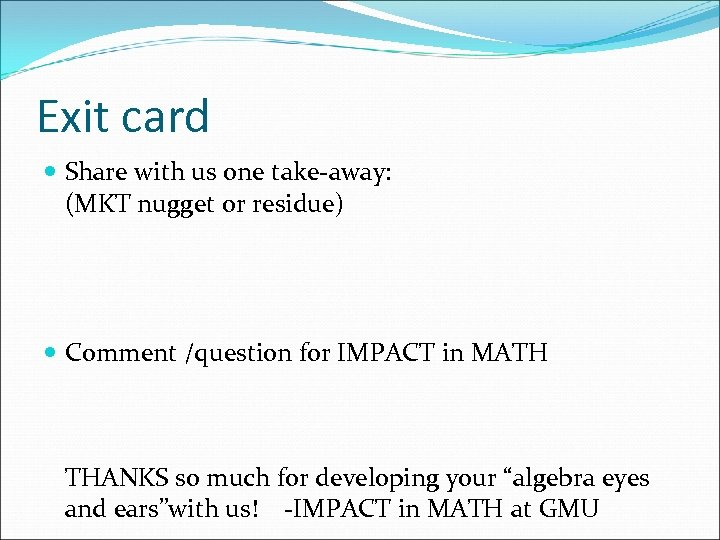Exit card Share with us one take-away: (MKT nugget or residue) Comment /question for IMPACT in MATH THANKS so much for developing your “algebra eyes and ears”with us! -IMPACT in MATH at GMU